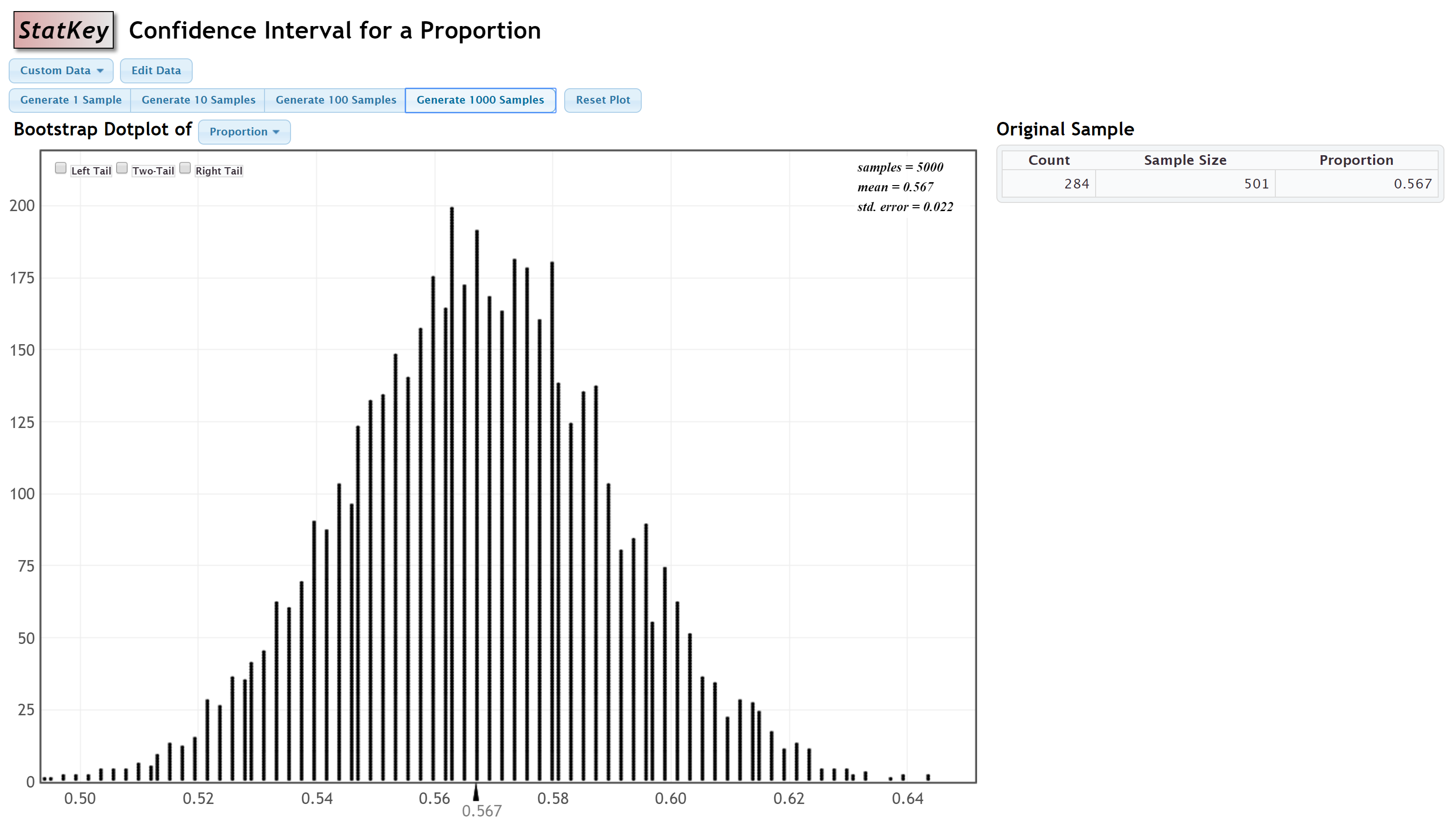# 7.4.2.3 - Example: 99% CI for Proportion of Women Students

Scenario: Data were collected from a representative sample of 501 World Campus STAT 200 students. In that sample, 284 students were women and 217 were not women. Construct a 99% confidence interval to estimate the proportion of all World Campus students who are women.

StatKey was used to construct a sampling distribution using bootstrapping methods:Because this distribution is approximately normal, we can approximate the sampling distribution using the z distribution. We will use the standard error, 0.022, from this distribution.

The original sample statistic was $$\widehat p =\frac{284}{501}=0.567$$.

We can find the $$z^*$$ multiplier by constructing a z distribution to find the values that separate the middle 99% from the outer 1%:The $$z^*$$ multiplier is 2.57583

Recall the general form of a confidence interval: sample statistic $$\pm$$ $$z^*$$ (standard error) where $$z^*$$ is the multiplier. So in this case we have...

$$0.567 \pm 2.57583 (0.022)$$

$$0.567 \pm 0.057$$

$$[0.510, 0.624]$$

I am 99% confident that the proportion of all World Campus students who are women is between 0.510 and 0.624